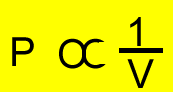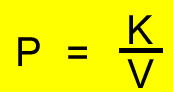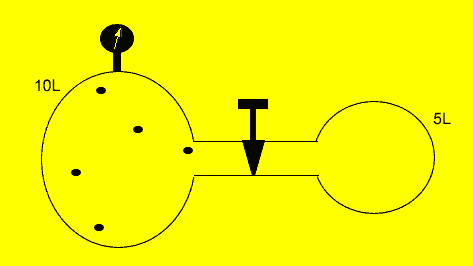Pressure and Volume Robert Boyle (1627 - 1691) investigated the relationship between pressure and volume of a fixed amount of gas at constant temperature. His investigations revealed that pressure and volume were inversely related. This realtionship is known as Boyle's Law.P= pressure V=volume We can conduct an experiment in the classroom to investigate this law but for now we will conduct a simple virtual experiment. Using 1kg bricks to apply pressure on the plunger of a glass syringe we will measure the resulting volume and plot the data on a set of axis.. Click to run the experiment. We can manipulate the expression on the right to form PV = K The product of pressure and volume is a constant for a fixed amount of gas at constant temperature. So if the pressure and volume of the gas changes the product of pressure and volume will be the same as shown by the expression below. P1V1 = P2V2OK so how do we use this formula? .Let's try some examples. We will use SI units so pressure will be kPa and volume is in litres.A fixed amount of gas was placed in a 10 litre vessel at a pressure of 101.3kPa. The tap was opened and the gas was allowed to flow freely into another 5 litre chamber as shown on the left. Calculate the final pressure inside the vessel if the temperature was kept constant. Since the amount of gas and the temperature were constant the expression below is true. P1V1 = P2V2 P1 = 101.3, V1 = 10L P2 = ?, V2 = 15L => 101.3 X 10 = P2 X 15 => 101.3 X 10 / 15 =P2 => 67.53kPa =P2

Home